# The Voting Classifier

## Introduction

The voting classifier is an ensemble learning method that combines several base models to produce the final optimum solution. The base model can independently use different algorithms such as KNN, Random forests, Regression, etc., to predict individual outputs. This brings diversity in the output, thus called Heterogeneous ensembling. In contrast, if base models use the same algorithm to predict separate outcomes, this is called Homogeneous ensembling.

The voting classifier is divided into hard voting and Soft voting.

### Hard voting

Hard voting is also known as majority voting. The base model's classifiers are fed with the training data individually. The models predict the output class independent of each other. The output class is a class expected by the majority of the models.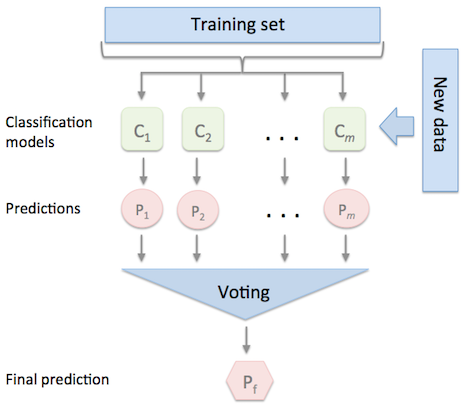Source: rasbt.github.io

In the above figure, Pf is the class predicted by the majority of the classifiers Cm.

### Soft voting

In Soft voting, Classifiers or base models are fed with training data to predict the classes out of m possible courses. Each base model classifier independently assigns the probability of occurrence of each type. In the end, the average of the possibilities of each class is calculated, and the final output is the class having the highest probability.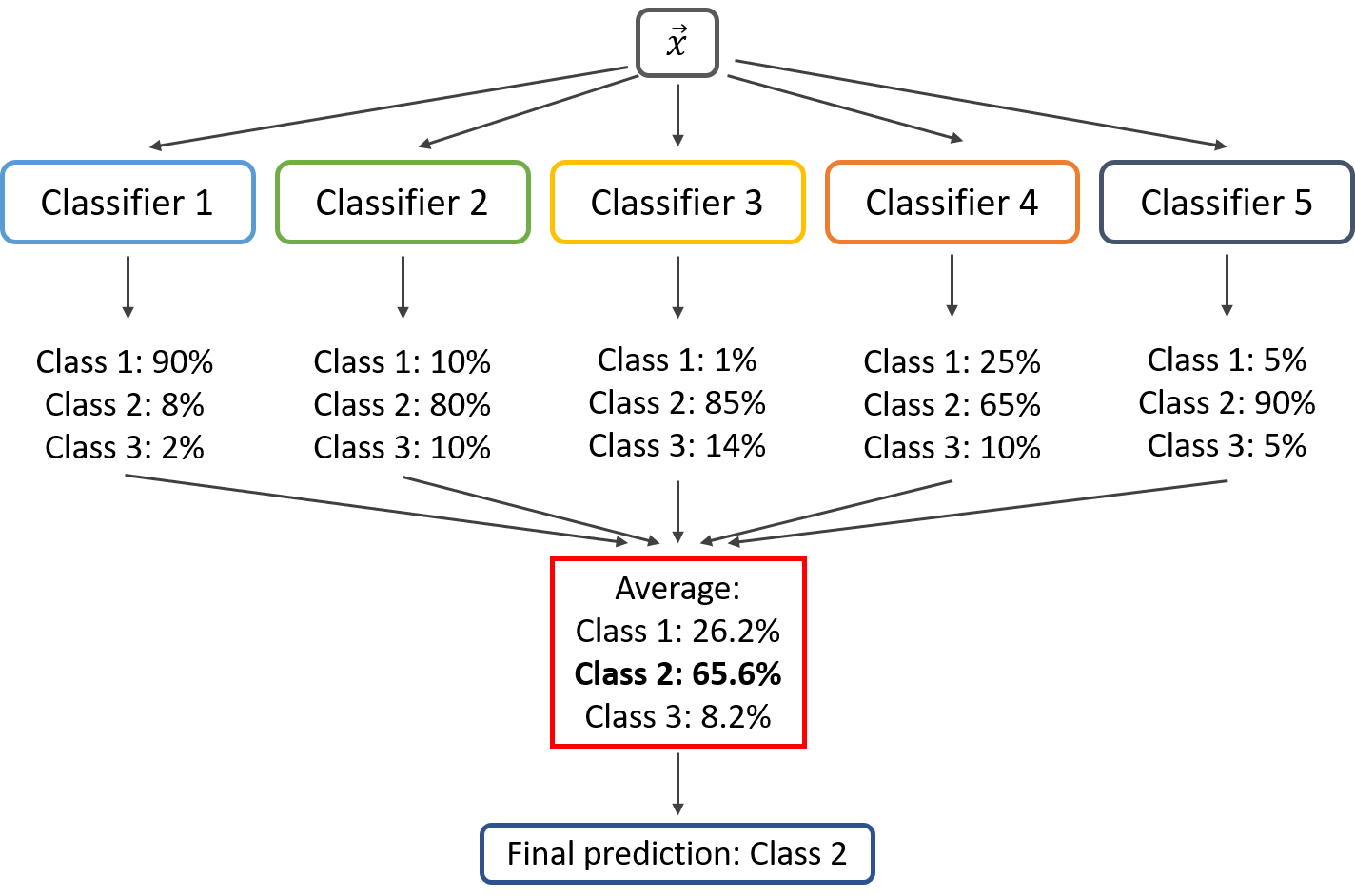Sourceiq.opengenus.org

## Implementation

Let’s implement the voting classifier.

I am importing the essential libraries and classifiers; SVC, Decision Tree, and Logistic Regression.

``````from sklearn.linear_model import LogisticRegression
from sklearn.svm import SVC
from sklearn.tree import DecisionTreeClassifier
from sklearn.metrics import accuracy_score``````

Let’s import the iris data set already available in SKlearn.

``from sklearn.datasets import load_iris``

``iris = load_iris()``

The iris dataset looks like this.

``print(iris.target_names)``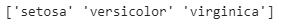``print(iris.target)``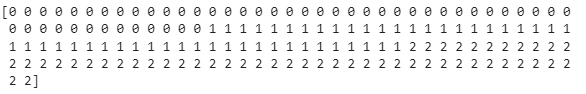Here 0 represents 'setosa', 1 means 'Versicolor,' and 2 illustrates Virginiaca.

I am separating the Independent and the target features.

``````X = iris.data[:, :4]
Y = iris.target``````

I am importing the model for training the data set.

``from sklearn.model_selection import train_test_split``

``X_train, X_test, y_train, y_test = train_test_split(X, Y, test_size = 0.25, random_state = 1)``

Ensembling the imported models

``````estimator = []
estimator.append(('LR', LogisticRegression(solver ='lbfgs', multi_class ='multinomial', max_iter = 200)))
estimator.append(('SVC', SVC(gamma ='auto', probability = True)))
estimator.append(('DTC', DecisionTreeClassifier()))``````

I am importing the voting classifier from SKlearn.

``from sklearn.ensemble import VotingClassifier``

Voting Classifier with hard voting

``````Hard = VotingClassifier(estimators = estimator, voting ='hard')
Hard.fit(X_train, y_train)
y_pred = Hard.predict(X_test)``````

using accuracy_score metric to predict the accuracy of Hard voting

``score = accuracy_score(y_test, y_pred)``
``print(score)``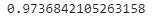The accuracy is 97%.

Voting Classifier with soft voting

``````Soft = VotingClassifier(estimators = estimator, voting ='soft')
Soft.fit(X_train, y_train)
y_pred = Soft.predict(X_test)``````

using accuracy_score metric to predict the accuracy of Soft voting

``Score = accuracy_score(y_test, y_pred)``
``print(Score)``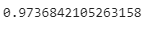The accuracy is 97%.

Q1) What is the feature of base models in the Voting classifier?
=> Base models in the Voting classifier have low variance, and thus overfitting is low.

Q2) Why do base models in Voting classifiers can't solve complex learning problems?
=> Base models in Voting classifiers have high bias, so they can't solve complex learning problems.

Q3) What are the main types of ensemble learning methods?

•      Bagging
•      Boosting
•      Stacking

Q4) What are the significant benefits of ensemble models?

•     Better prediction
•      More stable model

Q5) ___________ uses ensembles of ML models each capturing a specific subspace of predictor space.
=> Boosting

## Key Takeaways

There are various ensemble algorithms used in Machine learning. You can learn about them in more detail here. For more exciting machine learning algorithms, must visit: link

( Also checkBreast Cancer Classification using KNN )